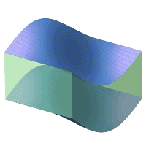Signal Processing - Maple Help

Home : Support : Online Help : System : Information : Updates : Maple 18 : Signal ProcessingSignal Processing

The Signal Processing package features tools for frequency domain analysis, windowing, signal generation & analysis, and more. In Maple 18, the Signal Processing toolkit has been expanded to include tools for visualizing the frequency contents of signals and expanded windowing functionality.

Compatibility note: For ease of use, the Engine subpackage of the SignalProcessing package has been removed, and the commands that were part of the Engine subpackage in Maple 17 are now present as top-level exports of the SignalProcessing package. Code and worksheets that referenced exports of SignalProcessing[Engine] will continue to work, but can now be updated to simply use exports of SignalProcessing itself.Spectrogram, Power Spectrum and Signal Plots

Updates include a new function that plots the Spectrogram of a signal, as well as plots for the Power Spectrum and the Signal Plot. By using the new ColorScheme option for Maple plots, you can control the color spread assigned to specific fractions of the given range.

 > $\mathrm{with}\left(\mathrm{SignalProcessing}\right):$

Example

This is a spectrogram of a male voice saying "MapleSim", recorded at 11 KHz.

 > $\mathrm{maplesim}≔\mathrm{FileTools}:-\mathrm{JoinPath}\left(\left[\mathrm{kernelopts}\left(\mathrm{datadir}\right),"audio","maplesim.wav"\right]\right):$$\mathrm{Spectrogram}\left(\mathrm{maplesim},\mathrm{colorscheme}=\left["zgradient",\left[\mathrm{white},\mathrm{LightSteelBlue},\mathrm{black}\right],\mathrm{markers}=\left[0,0.5,1\right]\right],\mathrm{fftsize}=256,\mathrm{size}=\left[400,400\right]\right)$Example

This is a spectrogram of a violin note played with vibrato, together with the power spectrum and signal plot. Note that the oscillations in frequency are typical of vibrato, and are visible above 7 kHz.

 >
 > $\mathrm{Spectrogram}\left(\mathrm{vibrato},\mathrm{colorscheme}=\left["zgradient",\left[\mathrm{white},\mathrm{LightBlue},\mathrm{red},\mathrm{black}\right],\mathrm{markers}=\left[0,0.5,0.75,1\right]\right],\mathrm{channel}=1,\mathrm{fftsize}={2}^{10},\mathrm{size}=\left[400,400\right],\mathrm{includepowerspectrum},\mathrm{includesignal}\right)$New Windowing Functions

Maple 18 offers more windowing functions, including Blackmann-Nuttall, Bohman windows, and more. Windows can also be applied to each window of data used to generate an FFT.

Example

 > $\mathrm{freq}≔5:$
 > $N≔{2}^{8}:$
 > $\mathrm{samplingRate}≔50:$
 > $\mathrm{data}≔\mathrm{Vector}\left(N,i\to \mathrm{sin}\left(\frac{2\cdot \mathrm{evalf}\left(\mathrm{Pi}\right)\cdot \mathrm{freq}\cdot i}{\mathrm{samplingRate}}\right)+\frac{\mathrm{rand}\left(\right)}{{10}^{12}},\mathrm{datatype}=\mathrm{float}\left[8\right]\right):\phantom{\rule[-0.0ex]{0.0em}{0.0ex}}\phantom{\rule[-0.0ex]{0.0em}{0.0ex}}\mathrm{dataWindow}≔\mathrm{BlackmanNuttallWindow}\left(\mathrm{data}\right):$
 > $\mathrm{Spectrogram}\left(\mathrm{dataWindow},\mathrm{samplerate}=\mathrm{samplingRate},\mathrm{includepowerspectrum},\mathrm{includesignal},\mathrm{fftsize}=128,\mathrm{colorscheme}=\left["zgradient",\left[\mathrm{SteelBlue},\mathrm{PaleGreen},\mathrm{red},\mathrm{Black}\right],\mathrm{markers}=\left[0,0.5,0.8,1\right]\right]\right)$New and Updated Applications

 Filtering Frequency Domain Noise Sunspot Periodicity Signal Generation Option Pricing with FFTsSee Also